Lumped Element Bias Tee

Click here to go to our page on self-resonant frequency in lumped elements

This page describes a simple method for developing a bias tee from lumped elements. Many low frequency amplifier MMICs (and wideband DC-coupled amplifiers) require a bias tee, but the app notes are a little vague on how to do it. Fear not, read this and you will be at least partially on your way!

Be sure to check out our discussion on self resonant frequency in lumped elements, also new for October 2011!

Only a nimrod would design a bias tee out of lumped elements and ignore the parasitics that cause self resonance. But every value of inductor and capacitor has an associated self resonance, which can be used to calculate the parasitics, but how do we fold the whole exercise together?

Fitting SRF to inductor and capacitor values

Below we have plotted some data on self-resonant frequency of inductors from a well known manufacturer ((just ask and we will tell you!) Turns out, if you plot the data on log scales, it pretty much falls into a straight line. We're not going to derive an explanation for that, we're engineers, not scientists.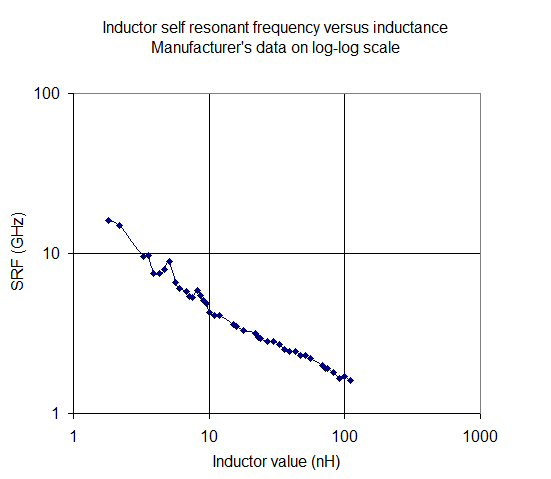The next step was to create a curve fit, simple once you know that the relationship is linear on log scales:

SRF=10^(-0.6log(L)+1.3)

Going to a linear scale, our curve fit and measured data are plotted below. It is not perfect, but good enough for initial designs that can use inductors from 1 to 100 nH.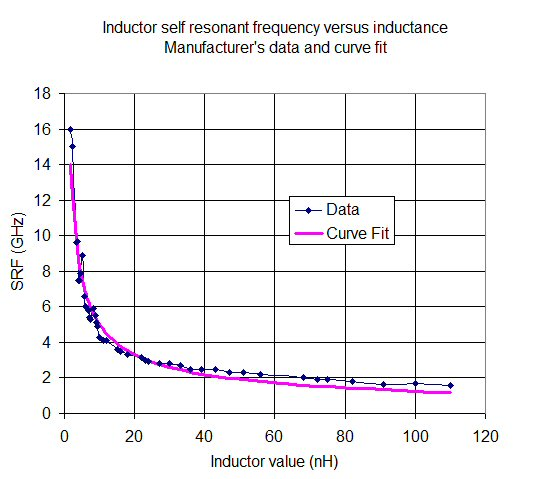Similarly, capacitors have a self-resonant frequency that fits a straight line on log-log scale. Below we have fit a model to a manufacturer's data, we will skip the step of plotting the data on the fit curve. Trust us....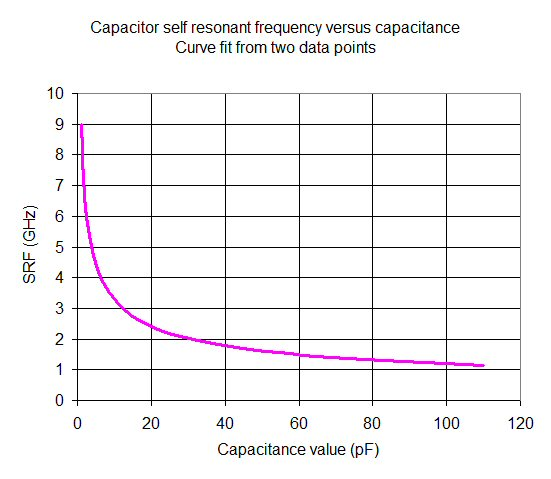Now we can create parameterized models for inductors and capacitors, that capture the parasitic elements that cause the first self resonance. Below is our inductor model. If you look on this page you will learn how to relate the self-resonant frequency to the parasitic capacitance C1 in the model. The resistance we left as a constant, you can play with it once you pick out an inductor that gives the bias tee the frequency response you want.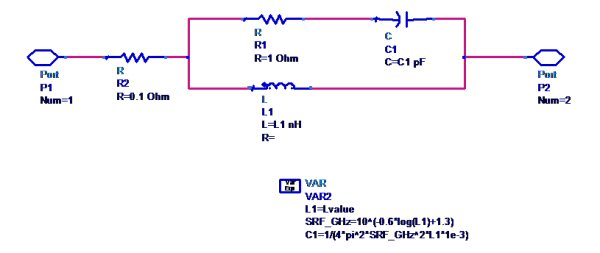And here is our capacitor model, with a similar conversion of the curve-fit SRF to the parasitic inductance. Again, we left resistance (ESR) as a constant that you can adjust later.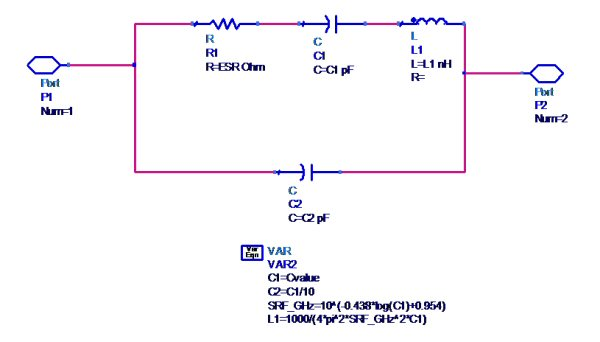Bias tee model and 1-10 GHz design

Here is the complete bias tee model, using two capacitors, and one inductor. Port 1 to 2 is the RF path, port 3 to 2 is the DC path. Capacitor X7 serves to block the DC signal from port 1. C1 is the DC blocking cap, C2 is the bypass cap on the bias line. This network can provide up to a decade of bandwidth. Some day maybe we will delve into the topic of increasing the bandwidth, or other considerations for linear operation.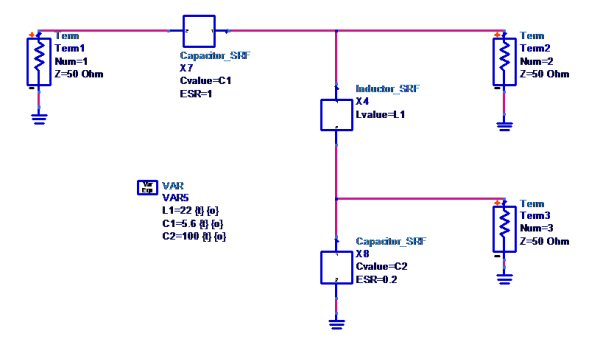We tweaked the values of L1, C1 and C2 until we obtained a bias tee that works from 12 to 10 GHz. The values of C1 and L1 are crucial to the RF path response; C2 can simply be maximized to obtaining the highest RF isolation down the bias arm.

By the way, we stuck with RETMA values for each component, and you should too. Lumped elements come in discrete values, which come with a tolerance.

Below is the passband of the RF path (port 1 to port 2). We have achieved less than 1 dB insertion loss from 1 to 10 GHz. If you believe that we will actually measure that we have a bridge to sell you, this model going to be optimistic until we go back and make sure we have the resistor values correct for the inductor and capacitor values.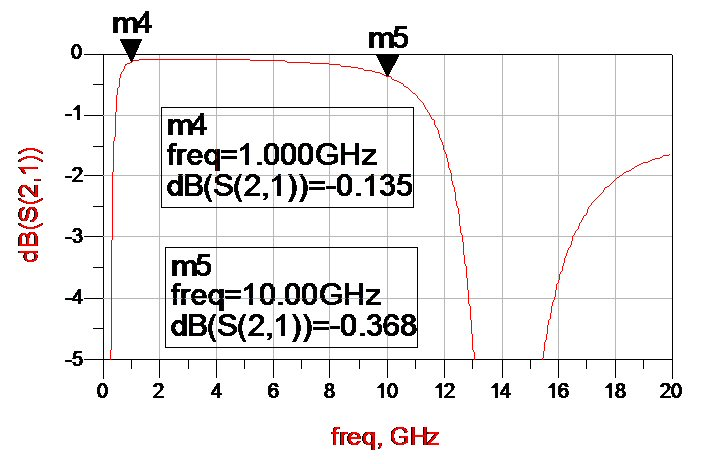Isolation of the DC path is shown below. We see better than 30 dB over most of the 1-10 GHz band.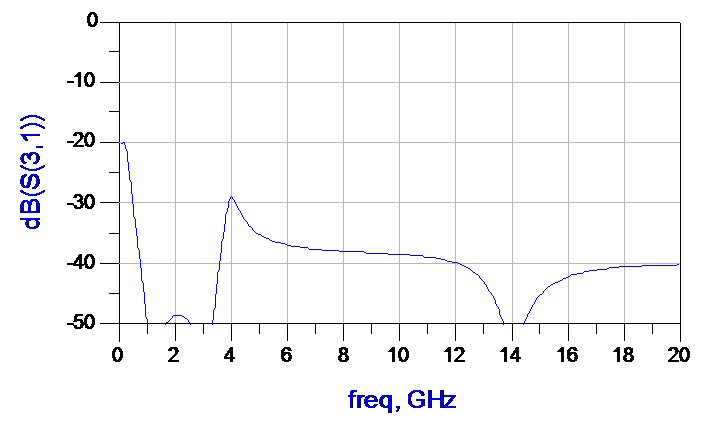Finally, here is return loss of the RF path at Port 1 (Port 2 is almost the same, but we should plot it too). We achieved 20 dB match across the band.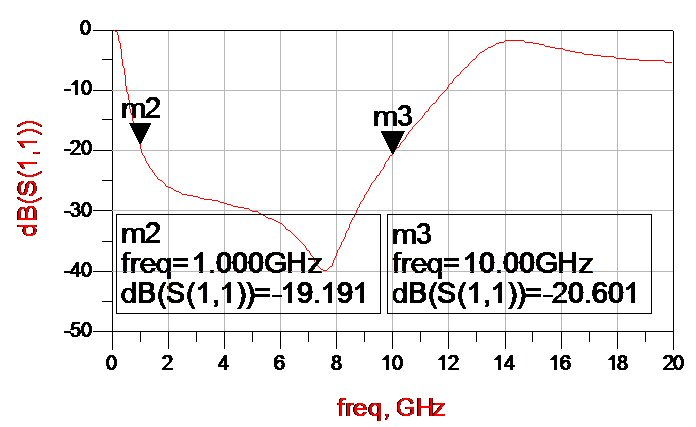In practice there are other considerations that must be taken into account that will reduce performance, such as layout parasitics (the pads that the lumped components mount to). Also you need to examine the effects of the component tolerances, perhaps with Monte Carlo simulation. But at least you have a head start!

Author : Unknown Editor